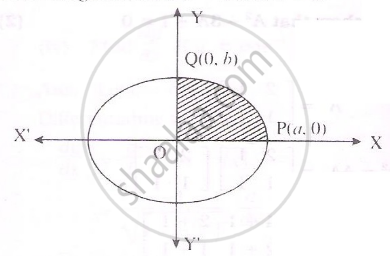# Find the area of elipse x^2/a^2+y^2/b^2=1 - Mathematics and Statistics

Find the area of elipse x^2/a^2+y^2/b^2=1

#### Solution

equation of elipse x^2/a^2+y^2/b^2=1

Clearly the area of elipse is 4 times the area of region OPQD as show in the figure.For the region.Limits of integration are x=0 and x=aFrom the elipse

y^2/b^2=1-x^2/a^2=(a^2-x^2)/a^2

therefore y^2=b^2/a^2(a^2-x^2)

y=+-b/a(a^2-x^2)^(1/2)

y=b/a(a^2-x^2)^(1/2)

We know

A=4int_0^1ydx

=int_0^ab/a(a^2-x^2)^(1/2)dx

=(4b)/a[x/2(a^2-x^2)^(1/2)+a^2/2sin^(-1)(x/a)]_0^a

=(4b)/a[a^2/2 pi/2-0]

=ab pi

Concept: Applications of Definite Integrals
Is there an error in this question or solution?
2014-2015 (March)

Share